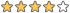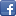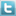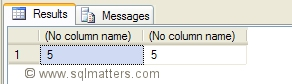Determining the length of a text column

 Category : Tips User Rating :Views :One Click Feedback

The length of a varchar column can be determined using the len() function, however this generates an error when used with the text datatype. Fortunately we can use the datalength() function to work out the length of a text field.

The following example shows how to use len() and datalength() to work out the length of a varchar and text column respectively.

I'll start be creating a test table with a varchar and a text column and populate both columns with the same string :

CREATE TABLE MyTable
(VarcharCol VARCHAR(100), TextCol TEXT)

INSERT MyTable (VarcharCol,TextCol)
VALUES ('Hello','Hello')
I can then check the length using len() and datalength() respectively :

SELECT  LEN(VarcharCol),DATALENGTH(TextCol)
FROM    MyTable
This produces the expected result of 5 for each column :There’s a couple of things to note here. Firstly Len() will strip out any trailing spaces in the string, but datalength() won’t. Datalength() actually works out the number of bytes rather than the number of characters which means that if the datatype is ntext (i.e. Unicode) the result will be twice the number of characters.

Related Articles

The following articles may also be of interest :